Disclaimer: This is an example of a student written essay.

Any scientific information contained within this essay should not be treated as fact, this content is to be used for educational purposes only and may contain factual inaccuracies or be out of date.

# Enthalpy of Combustion

 ✅ Paper Type: Free Essay ✅ Subject: Chemistry ✅ Wordcount: 5345 words ✅ Published: 16th Jan 2018

• Saran Singh Sound

Aim: To determine the enthalpy change of combustion of Ethanol (C2H5OH), Propanol (C3H7OH) and Butanol (C4H9OH).

Chemicals –

• Ethanol (C2H5OH)
• Propanol (C3H7OH)
• Butanol (C4H9OH)

Data Collection –

Table 1: List of apparatus and Least Count and Uncertainties of Measuring Instruments Used

 S. No. Instrument Unit Least Count Uncertainty 1. Digital Stopwatch Seconds 0.01s ±0.01s 2. Measuring Cylinder cm3 1cm3 ±0.5cm3 3. Digital Thermometer Celsius 0.1 ºC ±0.1 ºC 4. Digital Weighing Balance Grams 0.001g ±0.001g 5. Cardboard Lid — — — 6. Calorimeter — — — 7. Spirit Burner — — — 8. Wind Shield — — — 9. Clamp Stand — — —

Quantitative Data

• Temperature in degrees Celsius ± 0.1°C
• Time in seconds ± 0.01s

Qualitative Observation:

• It was observed that the flame on the spirit burner swayed due to wind interference. Also, the flame went off at few instances. When the flame was initially burnt its intensity was much greater than when it had burnt for a few seconds.

Section A – Finding the water equivalent of calorimeter

Table 2.2: Collected Data

 Time/t/s/±0.01s Temperature/T/°c/±0.1°c Trial 1 Trial 2 Trial 3 30.00 27.6 29.2 28.8 60.00 27.6 29.2 28.8 90.00 27.6 29.2 28.8 120.00 27.6 29.2 28.8 150.00 40.3 46.0 45.3 180.00 39.6 45.3 44.2 210.00 39.3 44.8 43.8 240.00 39.0 44.4 43.4 270.00 38.8 44.1 43.2 300.00 38.6 43.8 42.9 330.00 38.3 43.6 42.7 360.00 38.1 43.4 42.5 390.00 38.0 43.1 42.3 420.00 37.9 42.9 42.0 450.00 37.8 42.7 41.8 480.00 37.7 42.5 41.5 510.00 37.3 42.3 41.3 540.00 37.0 42.0 41.1 570.00 36.8 41.8 40.8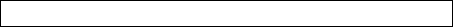Formulas:

Mass = No. of Moles x Molar MassMw x C (Thot – Tfinal) = Mw x C (Tfinal – Tcold) + Q (Tfinal – Tcold)â-²T = Initial Temperature – Extrapolation TemperatureEnthalpy Change = Mass x Specific Heat Capacity x â-²T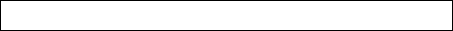Molar Mass of Ethanol = 46.068gMolar Mass of Propanol = 60.095gMolar Mass of Butanol = 74.122g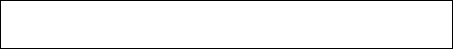Percentage Deviation =x100

Uncertainty Formulas:Error in mass of Water =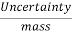x 100Error in temperature =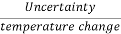x 100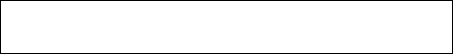Error in time =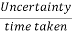x 100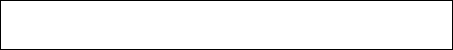Avg. Uncertainty =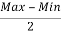Trial 1:

 Temperature of cold water/Tc 27.6 ± 0.1 ºC Temperature of hot water/Th 57.1 ± 0.1 ºC Mass of Water in Clorimeter/Mc 50.0 ± 0.5g Specific Heat Capacity 4.18 J.g‑1.ºC-1 Initial Temperature 27.6 ± 0.1ºC Extrapolation temperature/Tf 40.4 ± 0.1ºC Time at which Hot water was added 120.00 ± 0.01s

##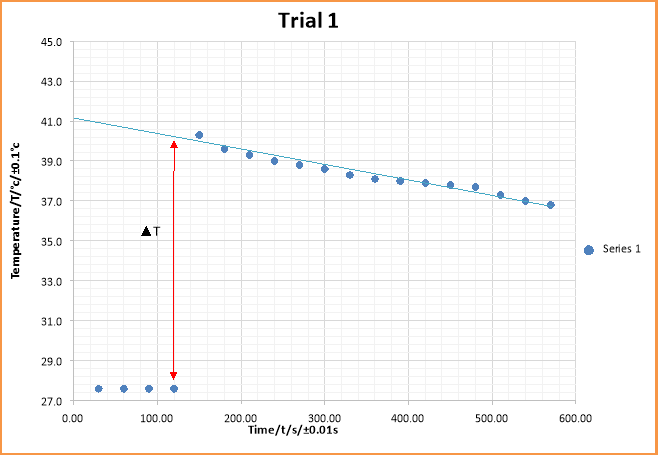Uncertainty of temperature is small and hence error bars cannot be seen on the graph

The graph is used to estimate the water equivalent of calorimeter. The gradient of the best fit line shows the rate of decrease of temperature.

Uncertainties:-

Error in mass of Water =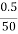x 100 = 1%

Error in Temperature of cold water/Tc =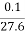x 100 = 0.4%

Error in Temperature of hot water/Th =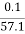x 100 = 0.2%

Error in Extrapolated Temperature/Tf =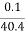x 100 = 0.2%

Calculation:-

Mw x C (Thot – Tfinal) = Mw x C (Tfinal – Tcold) + Q (Tfinal – Tcold)

... 50.0 x 4.18 (57.1 – 40.4) = 50.0 x 4.18 (40.4 – 27.6) + Q (40.4 – 27.6)

So, Q = 63.8g

Percentage Uncertainty for Q= 1% + (0.2% – 0.2%) = 1% + (0.2% -0.4%) + (0.2% – 0.4%)

... 1% + 0.6% = 1.6% Uncertainty

Absolute Uncertainty = 1.6% x 63.8 = ± 1g

So, Water equivalent of calorimeter = (64 ± 1)g

Note : There were three assumptions made during this experiment.

1. The Specific Heat Cpacity of the calorimeter is same as water
2. No Heat is lost to the surrounding
3. Mass of water is equal to volume of water

Trial 2:

 Temperature of cold water/Tc 30.1 ± 0.1 ºC Temperature of hot water/Th 63.6 ± 0.1 ºC Mass of Water in Clorimeter/Mc 50.0 ± 0.5g Specific Heat Capacity 4.18 J.g‑1.ºC-1 Initial Temperature 30.1 ± 0.1ºC Extrapolation temperature/Tf 45.6 ± 0.1ºC Time at which Hot water was added 120.00 ± 0.01s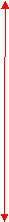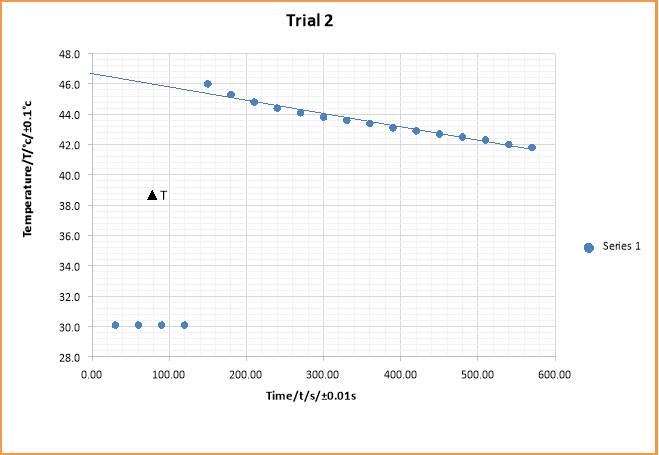Uncertainty of temperature is small and hence error bars cannot be seen on the graph

The graph is used to estimate the water equivalent of calorimeter. The gradient of the best fit line shows the rate of decrease of temperature.

Uncertainties:-

Error in mass of Water =x 100 = 1%

Error in Temperature of cold water/Tc =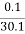x 100 = 0.3%

Error in Temperature of hot water/Th =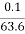x 100 = 0.2%

Error in Extrapolated Temperature/Tf =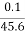x 100 = 0.2%

Calculation:-

Mw x C (Thot – Tfinal) = Mw x C (Tfinal – Tcold) + Q (Tfinal – Tcold)

... 50.0 x 4.18 (63.6 – 45.6) = 50.0 x 4.18 (45.6 – 30.1) + Q (45.6 – 30.1)

So, Q = 33.7g

Percentage Uncertainty for Q= 1% + (0.2% – 0.2%) = 1% + (0.2% – 0.3%) + (0.2% – 0.3%)

... 1% + 0.8% = 1.8% Uncertainty

Absolute Uncertainty = 1.8% x 33.7 = ± 0.6 g

So, Water equivalent of calorimeter = (33.7 ± 0.6)g

Note : There were three assumptions made during this experiment.

1. The Specific Heat Cpacity of the calorimeter is same as water
2. No Heat is lost to the surrounding
3. Mass of water is equal to volume of water

Trial 3:

 Temperature of cold water/Tc 29.8 ± 0.1 ºC Temperature of hot water/Th 62.6 ± 0.1 ºC Mass of Water in Clorimeter/Mc 50.0 ± 0.5g Specific Heat Capacity 4.18 J.g‑1.ºC-1 Initial Temperature 29.8 ± 0.1ºC Extrapolation temperature/Tf 44.6 ± 0.1ºC Time at which Hot water was added 120.00 ± 0.01s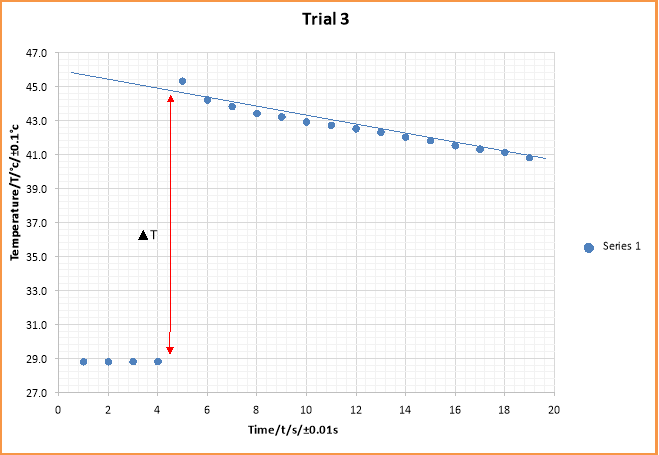Uncertainty of temperature is small and hence error bars cannot be seen on the graph

The graph is used to estimate the change in temperature of the displacement reaction between CuSO4 solution and Zinc (s) powder. The gradient of the best fit line shows the rate of decrease of temperature.

Uncertainties:-

Error in mass of Water =x 100 = 1%

Error in Temperature of cold water/Tc =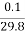x 100 = 0.3%

Error in Temperature of hot water/Th =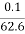x 100 = 0.2%

Error in Extrapolated Temperature/Tf =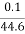x 100 = 0.2%

Calculation:-

Mw x C (Thot – Tfinal) = Mw x C (Tfinal – Tcold) + Q (Tfinal – Tcold)

... 50.0 x 4.18 (62.6 – 44.6) = 50.0 x 4.18 (44.6 – 29.8) + Q (44.6 – 29.8)

So, Q = 45.2g

Percentage Uncertainty for Q= 1% + (0.2% – 0.2%) = 1% + (0.2% – 0.3%) + (0.2% – 0.3%)

... 1% + 0.8% = 1.8% Uncertainty

Absolute Uncertainty = 1.8% x 45.2 = ± 0.8 g

So, Water equivalent of calorimeter = (45.2 ± 0.8)g

Note : There were three assumptions made during this experiment.

1. The Specific Heat Cpacity of the calorimeter is same as water
2. No Heat is lost to the surrounding
3. Mass of water is equal to volume of water

Average of all Trials:

Average Water Equivalent of calorimeter/Q =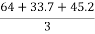= 47.6 g

Uncertainty for Average Water Equivalent of calorimeter/Q =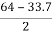= ± 15g

Therefore, Average Water Equivalent of calorimeter/Q = (48 ± 15)g

Section B – Finding the enthalpy of combustion of three alcohols.

Table 1.1: Collected Data

 Alcohols Trial Initial Mass of Spirit Lamp + Alcohol/Mi/g/±0.001g Final Mass of Spirit Lamp + Alcohol/Mf/g/±0.001g Initial Temperature of water/Ti/°C/±0.1°C Final Temperature of water/Ti/°C/±0.1°C Ethanol 1. 136.832 136.269 28.9 46.1 2. 136.269 135.934 29.0 47.7 3. 135.934 135.558 29.2 50.9 Propanol 1. 138.554 138.087 29.2 44.9 2. 138.087 137.812 29.0 45.9 3. 137.812 137.547 29.0 46.9 Butanol 1. 137.844 137.667 28.9 38.9 2. 137.667 137.367 29.4 45.2 3. 137.367 137.208 29.6 42.4

Note: Mass of Water was kept constant at 50.0 ± 0.5g

Enthalpy of combustion (ΔHØC) of ethanol :

Trial 1:

Mass of Ethanol lost = 136.832 – 136.269 = (0.563 ± 0.002)g

Temperature Change/ΔT = 28.9 – 46.1 = (-17.2 ± 0.2)°C

No of moles =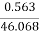= (0.0100 ± 0.0004)mol

ΔHØC =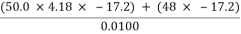= -442040 J.mol-1 =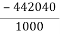= -442.040 kJ.mol-1

Uncertainties:

Error in mass of Water =x 100 = 1%

Error in No of Moles =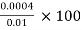= 4%

Error in Temperature Change/ ΔT =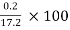= 1.2%

Error in Water equivalent of Calorimeter/Q =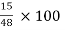= 31.3%

Percentage Uncertainty in ΔHØC = 2.2% + 32.5%) + 4% = 38.7%

Absolute Uncertainty = 38.7% x -442.040 = ± 171 kJ.mol-1

So, Enthalpy of Combustion of ethanol = (-442 ± 171 ) kJ.mol-1

Trial 2:

Mass of Ethanol lost = 136.269 – 135.934 = (0.335 ± 0.002)g

Temperature Change/ΔT = 28.9 – 47.7 = (-18.7 ± 0.2)°C

No of moles =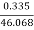= (0.0100 ± 0.0004)mol

ΔHØC =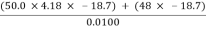= -480590 J.mol-1 =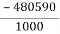= -480.590 kJ.mol-1

Uncertainties:

Error in mass of Water =x 100 = 1%

Error in No of Moles == 4%

Error in Temperature Change/ ΔT =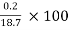= 1.2%

Error in Water equivalent of Calorimeter/Q == 31.3%

Percentage Uncertainty in ΔHØC = 2.2% + 32.5% + 4% = 38.7%

Absolute Uncertainty = 38.7% x -480.590 = ± 186 kJ.mol-1

So, Enthalpy of Combustion of ethanol = (-481 ± 186) kJ.mol-1

Trial 3:

Mass of Ethanol lost = 135.934 – 135.558 = (0.376 ± 0.002)g

Temperature Change/ΔT = 28.9 – 50.9 = (-21.7 ± 0.2)°C

No of moles =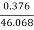= (0.0100 ± 0.0004) mol

ΔHØC =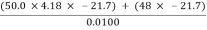= -557690 J.mol-1 =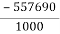= -557.690 kJ.mol-1

Uncertainties:

Error in mass of Water =x 100 = 1%

Error in No of Moles == 4%

Error in Temperature Change/ ΔT =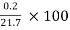= 1%

Error in Water equivalent of Calorimeter/Q == 31.3%

Percentage Uncertainty in ΔHØC = 2% + 32.3% + 4% = 38.3%

Absolute Uncertainty = 38.3% x -557.690 = ± 214 kJ.mol-1

So, Enthalpy of Combustion of ethanol = (-558 ± 214) kJ.mol-1

Average of all Trials:

Average Enthalpy of Combustion of ethanol =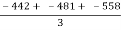= -494 kJ.mol-1

Uncertainty in Average Enthalpy of Combustion of ethanol =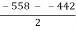= ± 58 kJ.mol-1

So, Average Enthalpy of Combustion of ethanol = (-494 ± 58) kJ.mol-1

Literature Value = -1368 kJ.mol -1

Therefore, Percentage Deviation = 63.9%

Enthalpy of combustion (ΔHØC) of propanol :

Trial 1:

Mass of Propanol lost = 138.554 – 138.087 = (0.467 ± 0.002)g

Temperature Change/ΔT = 28.9 – 44.9 = (-15.7 ± 0.2)°C

No of moles =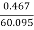= (0.0100 ± 0.0004) mol

ΔHØC =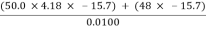= -403490 J.mol-1 =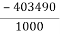= -403.490 kJ.mol-1

Uncertainties:

Error in mass of Water =x 100 = 1%

Error in No of Moles == 4%

Error in Temperature Change/ ΔT =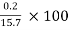= 1.2%

Error in Water equivalent of Calorimeter/Q == 31.3%

Percentage Uncertainty in ΔHØC = 2.2% + 32.5% + 4% = 38.7%

Absolute Uncertainty = 38.7% x -403.490 = ± 156 kJ.mol-1

So, Enthalpy of Combustion of propanol = (-403 ± 156) kJ.mol-1

Trial 2:

Mass of Propanol lost = 138.087 – 137.812 = (0.275 ± 0.002)g

Temperature Change/ΔT = 28.9 – 45.9 = (-16.9 ± 0.2)°C

No of moles =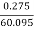= (0.0100 ± 0.0004)mol

ΔHØC =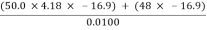= -434330 J.mol-1 = =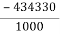= -434.330 kJ.mol-1

Uncertainties:

Error in mass of Water =x 100 = 1%

Error in No of Moles == 4%

Error in Temperature Change/ ΔT =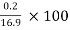= 1.2%

Error in Water equivalent of Calorimeter/Q == 31.3%

Percentage Uncertainty in ΔHØC = 2.2% + 32.5% + 4% = 38.7%

Absolute Uncertainty = 38.7% x -434.330 = ± 168 kJ.mol-1

So, Enthalpy of Combustion of propanol = (-434 ± 168) kJ.mol-1

Trial 3:

Mass of Propanol lost = 137.812 – 137.547 = (0.265 ± 0.002)g

Temperature Change/ΔT = 28.9 – 46.9 = (-17.9 ± 0.2)°C

No of moles =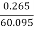= (0.0100 ± 0.0004)mol

ΔHØC =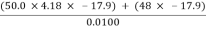= -460030 J.mol-1 =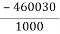= -460.030 kJ.mol-1

Uncertainties:

Error in mass of Water =x 100 = 1%

Error in No of Moles == 4%

Error in Temperature Change/ ΔT =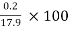= 1.2%

Error in Water equivalent of Calorimeter/Q == 31.3%

Percentage Uncertainty in ΔHØC = 2.2% + 32.5% + 4% = 38.7%

Absolute Uncertainty = 38.7% x -460.030 = ± 178 kJ.mol-1

So, Enthalpy of Combustion of propanol = (-460 ± 178) kJ.mol-1

Average of all Trials:

Average Enthalpy of Combustion of propanol =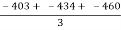= -432 kJ.mol-1

Uncertainty in Average Enthalpy of Combustion of propanol =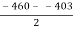= ± 29 kJ.mol-1

So, Average Enthalpy of Combustion of propanol = (-432 ± 29) kJ.mol-1

Literature Value = -2021 kJ.mol -1

Therefore, Percentage Deviation = 78.5%

Enthalpy of combustion (ΔHØC) of butanol :

Trial 1:

Mass of butanol lost = 137.844- 137.667 = (0.177 ± 0.002)g

Temperature Change/ΔT = 28.9 – 38.9 = (-10.0 ± 0.2)°C

No of moles =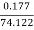= (0.0020 ± 0.0004)mol

ΔHØC =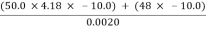= -1285000 J.mol-1 =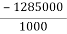= -1285.000 kJ.mol-1

Uncertainties:

Error in mass of Water =x 100 = 1%

Error in No of Moles =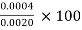= 0.2%

Error in Temperature Change/ ΔT = [Image_Link]https://s3-eu-west-1.amazonaws.com/aaimagestore/essays/

View all

## DMCA / Removal Request

If you are the original writer of this essay and no longer wish to have your work published on UKEssays.com then please:

Related Services

Related Lectures